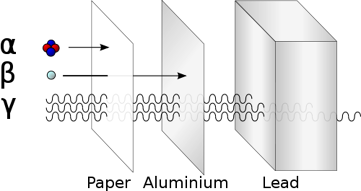## FANDOM

225 PagesAlpha (α). Beta (β). Gamma (γ).

## About

Ionizing radiation consists of particles or electromagnetic waves energetic enough to detach electrons from atoms or molecules, thus ionizing them. The degree and nature of such ionization depends on the energy of the individual particles or waves, and not on their number. An intense flood of particles or waves will not cause ionization if these particles or waves do not carry enough energy to be ionizing. Roughly speaking, particles or photons with energies above a few electron volts (eV) are ionizing. Ionization produces free radicals, which are atoms or molecules containing unpaired electrons, that tend to be especially chemically reactive due to their electronic structure. Fig 1. -- Alpha (α) radiation consists of a fast moving helium-4 (4He) nucleus and is stopped by a sheet of paper. Beta (β) radiation, consisting of electrons, is halted by an aluminum plate. Gamma (γ) radiation, consisting of energetic photons, is eventually absorbed as it penetrates a dense material such as brick walls. Neutron (n) radiation consists of free neutrons which are blocked using light elements, like hydrogen, which slow and/or capture them.

Examples of ionizing particles are alpha particles, beta particles, neutrons, and cosmic rays. The ability of an electromagnetic wave (photons) to ionize an atom or molecule depends on its frequency. Radiation on the short-wavelength end of the electromagnetic spectrum—high frequency ultraviolet, x-rays, and gamma rays—is ionizing. Lower-energy radiation, such as visible light, infrared, microwaves, and radio waves, are not ionizing.

The latter types of lower energy electromagnetic radiation may damage molecules, but the effect is generally indistinquishable from the effects of simple heating. Such heating does not produce free radicals until higher temperatures (for example, flame temperatures or "browning" temperatures, and above) are attained. In contrast, damage done by ionizing radiation produces free radicals, even at room temperatures and below, and production of such free radicals is the reason these and other ionizing radiations produce quite different types of chemical effects from (low-temperature) heating. Free radical production is also a primary basis for the particular danger to biological systems of relatively small amounts of ionizing radiation that are far smaller than needed to produce significant heating. Free radicals easily damage DNA, and ionizing radiation may also directly damage DNA by ionizing or breaking DNA molecules.

Ionizing radiation is ubiquitous in the environment, and also comes from radioactive materials, x-ray tubes, and particle accelerators. It is invisible and not directly detectable by human senses, so instruments such as Geiger counters are usually required to detect its presence. In some cases it may lead to secondary emission of visible light upon interaction with matter, as in Cherenkov radiation and radioluminescence. It has many practical uses in medicine, research, construction, and other areas, but presents a health hazard if used improperly. Exposure to radiation causes damage to living tissue, and high doses can result in mutation, radiation sickness, cancer, and death.

## Units of measure

The units used to measure ionizing radiation are rather complex. The ionizing effects of radiation are measured by units of exposure:

• The coulomb per kilogram (C/kg) is the SI unit of ionizing radiation exposure, and measures the amount of radiation required to create 1 coulomb of charge of each polarity in 1 kilogram of matter.
• The roentgen (R) is an older traditional unit that is almost out of use, which represented the amount of radiation required to liberate 1 esu of charge of each polarity in 1 cubic centimeter of dry air. 1 Roentgen = 2.58×10−4 C/kg

However, the amount of damage done to matter (especially living tissue) by ionizing radiation is more closely related to the amount of energy deposited rather than the charge. This is called the absorbed dose.

• The gray (Gy), with units J/kg, is the SI unit of absorbed dose, which represents the amount of radiation required to deposit 1 joule of energy in 1 kilogram of any kind of matter.
• The rad (radioactivity absorbed dose), is the corresponding traditional unit which is 0.01 J deposited per kg. 100 rad = 1 Gy.

Equal doses of different types or energies of radiation cause different amounts of damage to living tissue. For example, 1 Gy of alpha radiation causes about 20 times as much damage as 1 Gy of x-rays. Therefore the equivalent dose was defined to give an approximate measure of the biological effect of radiation. It is calculated by multiplying the absorbed dose by a weighting factor WR which is different for each type of radiation (see above table).

• The sievert (Sv) is the SI unit of equivalent dose. Although it has the same units as grays, J/kg, it measures something different. It is the dose of a given type of radiation in Gy that has the same biological effect on a human as 1 Gy of x-rays or gamma radiation.
• The rem (Roentgen equivalent man) is the traditional unit of equivalent dose. 1 sievert = 100 rem. Because the rem is a relatively large unit, typical equivalent dose is measured in millirem (mrem), 10−3 rem, or in microsievert (μSv), 10−6 Sv. 1 mrem = 10 μSv.
• A unit sometimes used for low level doses of radiation is the BRET (Background Radiation Equivalent Time). This is the number of days of an average person's background radiation exposure the dose is equivalent to. This unit is apparently not standardized, and depends on the value used for the average background radiation dose. Using the 2000 UNSCEAR value (below), one BRET unit is equal to about 6.6 μSv.

For comparison, the average 'background' dose of natural radiation received by a person is around 3.6 mSv (360 mrem) per year. The lethal full-body dose of radiation for a human is around 4 - 5 Sv (400 - 500 rem)